# Joint Cost Allocation Methods### Written byTrue Tamplin, BSc, CEPF®

Updated on March 29, 2022

Several methods are available to allocate the joint production cost incurred up to the split-off point. These methods are:

• Quantitative method (or physical unit method): This is based on a physical measurement unit such as weight
• Weighted average method: This is based on a predetermined standard or index of production
• Market value method (or sales value method): This is based on the relative market values of the individual product

### Quantitative Method

This method apportions the total production cost to the various products on the basis of a predetermined standard or index of production. An average unit cost is obtained by dividing the total number of units produced into the total joint production cost.

As long as all units produced are measured in terms of the same unit and do not differ substantially, this method is simple and easy to apply. However, when the units produced are not measured in like terms, the method cannot be applied.

Example

Joint products A, B, C, and D are produced at a total joint production cost of \$120,000. The following quantities are produced: A, 20,000 units; B, 15,000 units; C, 10,000 units; and D, 15,000 units.

Required: Calculate the joint production cost allocation using the quantitative method.

Solution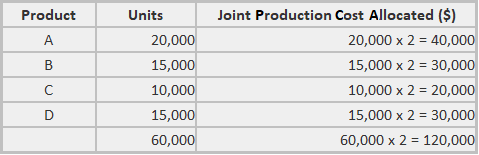Working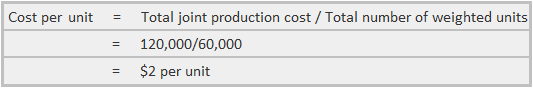Companies that use this method argue that all of the products produced using the same process should receive a proportionate share of the total joint production cost (based on the number of units produced).

### Weighted Average Method

In many industries, the quantitative method does not give a satisfactory answer to the joint cost apportionment problem.

For this reason, weight factors are often assigned to each unit based on unit size, manufacturing difficulty, manufacturing time, differences in the type of labor employed, the amount of material used, and so on.

Finished production of every kind is multiplied by weight factors to apportion the total joint cost to individual units.

Example

Using figures from the previous example, the following weight factors have been assigned to the four products as follows:

• Product A, 3 points
• Product B, 12 points
• Product C, 13.5 points
• Product D, 15 points

Required: Calculate the joint production cost allocation using the weighted average method.

Solution

The joint production cost allocation would result in the following values: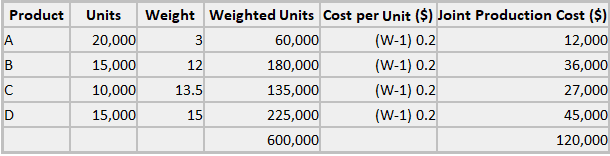Working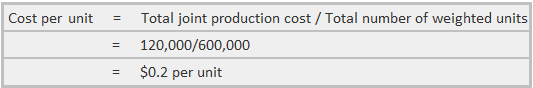### Market Value Method

This method is popular due to the argument that a product’s market value reflects the cost incurred to produce it. The contention is that if one product sells for more than another, it is because it cost more to produce.

Therefore, the way to allocate the joint cost is based on the respective market values of the items produced. The method is really a weighted market value basis using the total market or sales value of each unit (quantity sold multiplied by the unit sales price).

Example Joint products A, B, C, and D are produced at a total joint production cost of \$120,000. The following quantities are produced: A, 20,000 units; B, 15,000 units; C, 10,000 units; and D, 15,000 units.

Additionally, product A sells for \$0.25, B for \$3.00, C for \$3.50, and D for \$5.00. These prices are market or sales values for the products at the split-off point (i.e., it is assumed that they can be sold at the point).

Management may have decided, however, that it is more profitable to process certain products further before they are sold.

Nevertheless, this condition does not destroy the usefulness of the sales value at the split-off point for the allocation of the joint production cost.

Required: Calculate the joint production cost allocation using the market value method.

Solution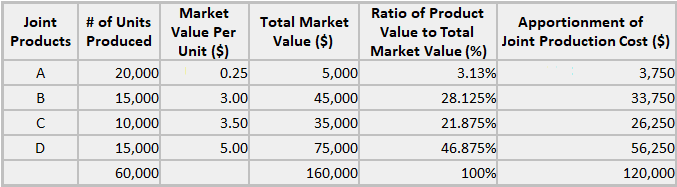## Joint Cost Allocation Methods FAQs